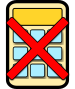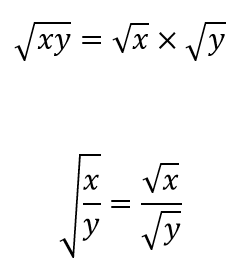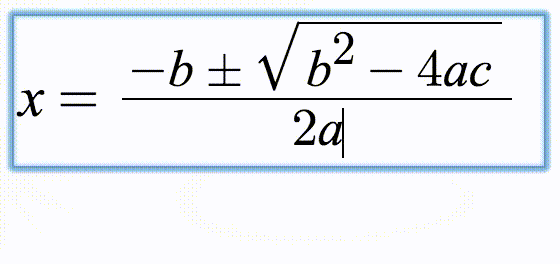# Surds

## A self-marking exercise on calculating, simplifying and manipulating surds (radicals)

##### Menu  Level 1Level 2Level 3Level 4Level 5Level 6Level 7Level 8ExamHelpWithout using a calculator simplify the following. You can use the √ button to insert the root or radical symbol. You can earn a trophy if you get at least 9 questions correct and you do this activity online. Click the Help tab above for more information.

 1 $$\sqrt{4}$$ ☐ ☐ ✓ ✗ 2 $$\sqrt{18}$$ ☐ ☐ ✓ ✗ 3 $$\sqrt{27}$$ ☐ ☐ ✓ ✗ 4 $$\sqrt{67}$$ ☐ ☐ ✓ ✗ 5 $$\sqrt{245}$$ ☐ ☐ ✓ ✗ 6 $$\sqrt{54}$$ ☐ ☐ ✓ ✗ 7 $$\sqrt{343}$$ ☐ ☐ ✓ ✗ 8 $$\sqrt{155}$$ ☐ ☐ ✓ ✗ 9 $$\sqrt{36}$$ ☐ ☐ ✓ ✗ 10 $$\sqrt{360}$$ ☐ ☐ ✓ ✗ 11 $$\sqrt{99}$$ ☐ ☐ ✓ ✗ 12 $$\sqrt{187}$$ ☐ ☐ ✓ ✗
Check

This is Surds level 1. You can also try:
Level 2 Level 3 Level 4 Level 5 Level 6 Level 7 Level 8

## Instructions

Try your best to answer the questions above. Type your answers into the boxes provided leaving no spaces. As you work through the exercise regularly click the "check" button. If you have any wrong answers, do your best to do corrections but if there is anything you don't understand, please ask your teacher for help.

When you have got all of the questions correct you may want to print out this page and paste it into your exercise book. If you keep your work in an ePortfolio you could take a screen shot of your answers and paste that into your Maths file.

## Transum.org

This web site contains over a thousand free mathematical activities for teachers and pupils. Click here to go to the main page which links to all of the resources available.## More Activities:

Mathematicians are not the people who find Maths easy; they are the people who enjoy how mystifying, puzzling and hard it is. Are you a mathematician?

Comment recorded on the 24 May 'Starter of the Day' page by Ruth Seward, Hagley Park Sports College:

"Find the starters wonderful; students enjoy them and often want to use the idea generated by the starter in other parts of the lesson. Keep up the good work"

Comment recorded on the 28 May 'Starter of the Day' page by L Smith, Colwyn Bay:

"An absolutely brilliant resource. Only recently been discovered but is used daily with all my classes. It is particularly useful when things can be saved for further use. Thank you!"

Each month a newsletter is published containing details of the new additions to the Transum website and a new puzzle of the month.

The newsletter is then duplicated as a podcast which is available on the major delivery networks. You can listen to the podcast while you are commuting, exercising or relaxing.

Transum breaking news is available on Twitter @Transum and if that's not enough there is also a Transum Facebook page.

##### Featured ActivityThe Transum Newsletter for October 2023 has just been published. Click on the image above to read about the latest developments on this site and try to solve the puzzle of the month. You can read the newsletter online or listen to it by downloading the podcast.

There are answers to this exercise but they are available in this space to teachers, tutors and parents who have logged in to their Transum subscription on this computer.

A Transum subscription unlocks the answers to the online exercises, quizzes and puzzles. It also provides the teacher with access to quality external links on each of the Transum Topic pages and the facility to add to the collection themselves.

Subscribers can manage class lists, lesson plans and assessment data in the Class Admin application and have access to reports of the Transum Trophies earned by class members.

Subscribe

## Go Maths

Learning and understanding Mathematics, at every level, requires learner engagement. Mathematics is not a spectator sport. Sometimes traditional teaching fails to actively involve students. One way to address the problem is through the use of interactive activities and this web site provides many of those. The Go Maths page is an alphabetical list of free activities designed for students in Secondary/High school.

## Maths Map

Are you looking for something specific? An exercise to supplement the topic you are studying at school at the moment perhaps. Navigate using our Maths Map to find exercises, puzzles and Maths lesson starters grouped by topic.

## Teachers

If you found this activity useful don't forget to record it in your scheme of work or learning management system. The short URL, ready to be copied and pasted, is as follows:

Alternatively, if you use Google Classroom, all you have to do is click on the green icon below in order to add this activity to one of your classes.

It may be worth remembering that if Transum.org should go offline for whatever reason, there is a mirror site at Transum.info that contains most of the resources that are available here on Transum.org.

When planning to use technology in your lesson always have a plan B!

Sunday, March 15, 2020

Do you have any comments? It is always useful to receive feedback and helps make this free resource even more useful for those learning Mathematics anywhere in the world. Click here to enter your comments.For All:

Scan the QR code below to visit the online version of this activity.https://Transum.org/go/?Num=3

## Description of Levels

Close

Level 1 - Simplifying surds

Level 2 - Simplifying the product of two surds

Level 3 - Simplifying the product of integers and surds

Level 4 - Simplifying the sum of integers and surds

Level 5 - Simplifying fractions containing surds

Level 6 - Rationalising the denominator of a fraction

Level 7 - Miscellaneous questions involving surds

Level 8 - Finding the conjugate surd to rationalise the denominator

Paper Surprising Perimeter - A wonderful surds problem with a surprising result.

Exam Style Questions - A collection of problems in the style of GCSE or IB/A-level exam paper questions (worked solutions are available for Transum subscribers).

More on this topic including lesson Starters, visual aids, investigations and self-marking exercises.

Answers to this exercise are available lower down this page when you are logged in to your Transum account. If you don’t yet have a Transum subscription one can be very quickly set up if you are a teacher, tutor or parent.

There are three statements referring to surds in the English National Curriculum:

• Pupils should be taught to calculate exactly with fractions, {surds} and multiples of π ; {simplify surd expressions involving squares [for example √12 = √(4 × 3) = √4 × √3 = 2√3] and rationalise denominators}
• Pupils should be taught to simplify and manipulate algebraic expressions including those involving surds ...
• Pupils should be taught to recognise and use sequences ... and simple geometric progressions (rn where n is an integer, and r is a positive rational number {or a surd})

## Help

The key properties you need are:## Examples

$$\sqrt{80} = \sqrt{4\times4\times5} = \sqrt{4}\times\sqrt{4}\times\sqrt{5} = 2\times2\times\sqrt{5} = 4\sqrt{5}$$

$$\sqrt{8}\times\sqrt{12} = \sqrt{4\times2}\times\sqrt{4\times3} = 2\times\sqrt{2}\times2\times\sqrt{3} = 4\sqrt{6}$$

$$(5+\sqrt{7})(5-\sqrt{7}) = 25+5\sqrt{7}-5\sqrt{7}-7 = 25-7 = 18$$

Answers should be given in their simplest form. For example:

$$\sqrt{3}-1$$ is simpler than $$-1+\sqrt{3}$$ as it uses less symbols.

$$\dfrac{5-\sqrt{3}}{2}$$ is simpler than $$\dfrac{\sqrt{3}-5}{-2}$$ as it uses less symbols.

### Typing Mathematical Notation

These exercises use MathQuill, a web formula editor designed to make typing Maths easy and beautiful. Watch the animation below to see how common mathematical notation can be created using your keyboard.Don't wait until you have finished the exercise before you click on the 'Check' button. Click it often as you work through the questions to see if you are answering them correctly. You can double-click the 'Check' button to make it float at the bottom of your screen.

Answers to this exercise are available lower down this page when you are logged in to your Transum account. If you don’t yet have a Transum subscription one can be very quickly set up if you are a teacher, tutor or parent.

Close